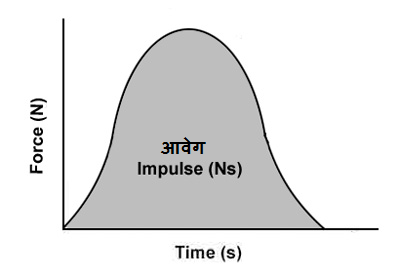# Impulse and Impulse-Momentum theorem

### Impulse and Impulse-Momentum theorem

Change in the velocity of an object can be made in two ways-

• Applying a large force on the object for a short time.
• By applying a smaller magnitude of force for long time.

Thus we can say that the change in velocity in an object is proportional to the product of force applied and the time duration.

Impulse: – When a force of greater magnitude is applied on an object for a short time (for example, hitting the ball with the bat, hitting the nail with the hammer), the product of the force and time difference is called the impulse of the force.

This is the vector quantity and it is expressed by the symbol I.

The direction of impulse is in the direction of force.

Unit of impulse: –

Newton-second (N-s)

Formula of impulse: –

If a force F is acting on an object for a short time dt, then the impulse of this force

dI = F dt

Total impulse  I = ∫Fdt

= Area of ​​the graph between force and time##### (IMPULSE-MOMENTUM THEOREM):-

From Newton’s Second Law of Motion… ..

F = dP/dt

∴ Fdt = dP

⇒ Fdt = ∫dP

⇒ I = P2 – P1

This implies that the Impulse of a force is equal to the change in momentum of the body due to that force. This is the impulse-momentum theorem.